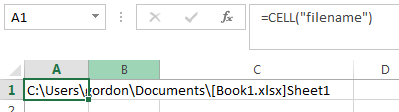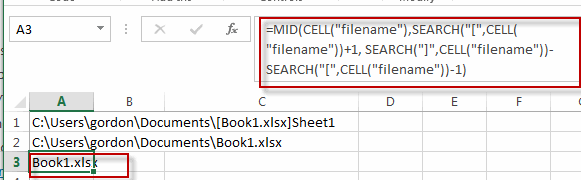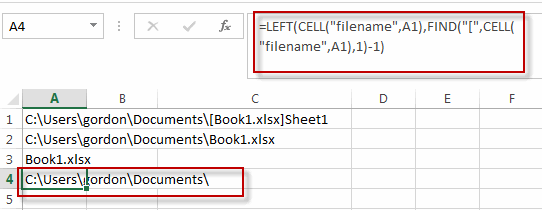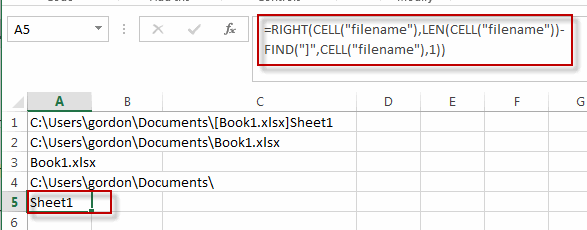# Insert The File Path and Filename into Cell

This post will guide you how to insert the file path and filename into a cell in Excel. Or how to add a file path only in a specified cell with a formula in Excel. Or how to add the current workbook name into a cell in Excel. How do I insert the current worksheet name into a Cell with a formula.

## Insert File Path and Filename into Cell

If you want to insert a file path and filename into a cell in your current worksheet, you can use the CELL function to create a formual. Just like this:

`=CELL("filename")`

Type this formula into a blank cell, and then press Enter key in your keyboard.This fromula will return the entire path, filename, and tab name of the current worksheet.

If you want to strip out the brackets and the sheet name, you can use the following formula based on the SUBSTITUTE function, the LEFT function, the CELL function and the FIND function:

`=SUBSTITUTE(LEFT(CELL("filename"),FIND("]",CELL("filename"))-1),"[","")`

## Insert Filename Only into Cell

If you only want to insert the workbook name into a cell, you can use a formula based on the MID function, the SEARCH function, and the CELL function. Just like this:

`=MID(CELL("filename"),SEARCH("[",CELL("filename"))+1, SEARCH("]",CELL("filename"))-SEARCH("[",CELL("filename"))-1)`

Type this formula into a blank cell and then press Enter key.You will see this formula will return only the current workbook name in Cell.

## Insert Filepath Only into Cell

If you just want to insert the File Path into a cell, you can create a formula based on the LEFT function, the Cell function,and the FIND function. Just like this:

`=LEFT(CELL("filename",A1),FIND("[",CELL("filename",A1),1)-1)`

Type this formula into a blank cell and then press Enter key to apply this formula.This formula will return only the File path of the current workbook.

## Insert Worksheet Name into Cell

If you want to get the worksheet name only and then insert it into one cell, you can create a formula based on the RIGHT function, the LEN function, the CELL function and the FIND function. Just like this:

`=RIGHT(CELL("filename"),LEN(CELL("filename"))-FIND("]",CELL("filename"),1))`

Type this formula into a blank cell and then press Enter key to apply this formula.This formula will return only the current worksheet name in a cell.

### Related Functions

• Excel Find function
The Excel FIND function returns the position of the first text string (substring) from the first character of the second text string.The FIND function is a build-in function in Microsoft Excel and it is categorized as a Text Function.The syntax of the FIND function is as below:= FIND  (find_text, within_text,[start_num])…
• Excel MID function
The Excel MID function returns a substring from a text string at the position that you specify.The syntax of the MID function is as below:= MID (text, start_num, num_chars)…
• Excel CELL function
The Excel CELL function returns information about the formatting, location, size, or contents of a cell.The syntax of the CELL function is as below:= CELL (info_type,[reference])…
• Excel Substitute function
The Excel SUBSTITUTE function replaces a new text string for an old text string in a text string.The syntax of the SUBSTITUTE function is as below:= SUBSTITUTE  (text, old_text, new_text,[instance_num]) ….
• Excel LEFT function
The Excel LEFT function returns a substring (a specified number of the characters) from a text string, starting from the leftmost character.The LEFT function is a build-in function in Microsoft Excel and it is categorized as a Text Function.The syntax of the LEFT function is as below:= LEFT(text,[num_chars])…
• Excel SEARCH function
The Excel SEARCH function returns the number of the starting location of a substring in a text string.The syntax of the SEARCH function is as below:= SEARCH  (find_text, within_text,[start_num])…
• Excel LEN function
The Excel LEN function returns the length of a text string (the number of characters in a text string).The LEN function is a build-in function in Microsoft Excel and it is categorized as a Text Function.The syntax of the LEN function is as below:= LEN(text)…
• Excel RIGHT function
The Excel RIGHT function returns a substring (a specified number of the characters) from a text string, starting from the rightmost character.The syntax of the RIGHT function is as below:= RIGHT (text,[num_chars])…